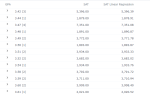# MLLIB.LINEARREG(fit_intercept, standardize, columns)

The MLLIB.LINEARREG function performs a linear regression to predict values of the dependent variable (first column) from independent variables (other columns).

Linear regression can be used when you have a linear relationship between the two continuous variables and no significant outliers. For example, you could use linear regression to understand whether the exam score can be predicted based on the preparation time. The variable to predict is the dependent variable (exam score), and the number of hours spent studying is the independent variable.

###### Parameters
• fit_intercept – Specifies whether intercept should be fitted, Boolean (for example, True).

• standardize – Specifies whether input variables should be standardized, Boolean (for example, True).

• columns – Dataset columns or custom calculations in the following order: dependent variable, independent variable.

Example: MLLIB.LINEARREG(True, True, [SAT], [GPA]).

###### Input data
• The size of input data is not limited.
• Input data without missing values.
• Character variables are transformed into numeric with label encoding.
###### Result
• A column of predicted values of the target variable, float.
###### Example

In the Table widget, we added a calculation with the MLLIB.LINEARREG(True, True, [SAT], [GPA]) function. The new column contains predicted SAT scores based on the corresponding GPA (Grade Point Average).For the whole list of algorithms, see Data science built-in algorithms.